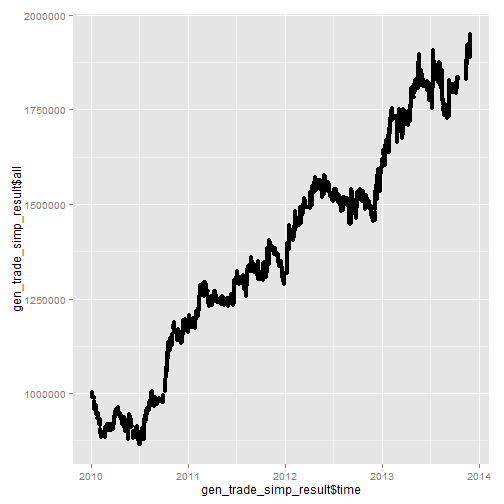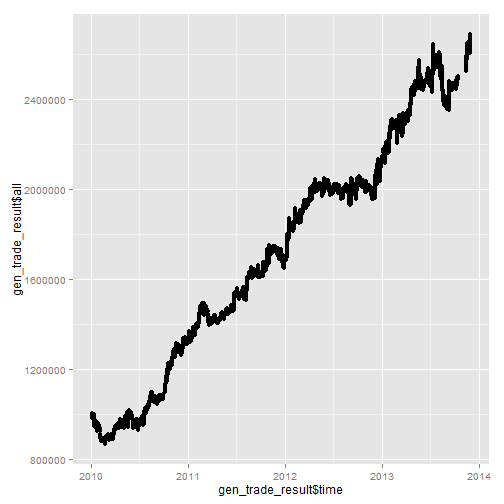Fleeting YearsTime goes.

Dual Thrust is a very simple but seemly effective strategy in quantitative investment.

Attention: I am NOT responsible for ANY of your loss!

strategy

1. After the close of first day, let m = max(FirstDayHighestPrice-FirstDayClosePrice, FirstDayClosePrice-FirstDayLowestPrice), then let SecondDayTrigger1 = m * k1, SecondDayTrigger2 = m * k2. SencondDayTrigger1 and SecondDayTrigger2 are called trigger values.

2. In the second day, note down the SecondDayOpenPrice. Once the price is higher than SecondDayOpenPrice + SecondDayTrigger1, buy. And once the price is lower than SecondDayOpenPrice - SecondDayTrigger2, sell short.

3. This system is a reversal system. Say, Once the price is higher than SecondDayOpenPrice + SecondDayTrigger1, and buy two shares if having a short shares. And once the price is lower than SecondDayOpenPrice - SecondDayTrigger2, short sell two shares if having a long share. (TODO: precise translation in English. 如果在价格超过（开盘＋触发值1）时手头有一手空单，则买入两手。如果在价格低于（开盘－触发值2）时手上有一手多单，则卖出两手。)

keypoints

This strategy is a super-easy one. It’s possible to build an automated trading system to do all the jobs. But of course there are some risks. For example, how to choose k1 and k2 and how they influence the result are not clear for me. Moreover, sometimes the stock runs vibrately, then the strategy will cause loss in the unexpected way. Last but not least, no stop-loss order is included in this strategy. It’s guessed (by me) that reducing k2 may stop loss to some extend.

simulation

If you want to reproduce the result or do some further research, you can download the min1_sh000300.csv and some other data from this page .

And possible-updated code for this project is on Github . Of course forks and pull requests are welcome.

I choose the Shanghai Shenzhen CSI 300 Index (000300.SS) to run the simulation. I acquired min1_sh000300.csv, the high frequency (every-minute-level) index price of CSI300 from 2010-01-01 to 2013-11-30, with some days lost.

There are some assumptions and limitations in the simulation. I assume I have 1,000,000 (one million) yuan cash available (WOW), and I cannot borrow shares more than those valued as 50% of one million. And I started to apply the strategy from 2010-01-01 to 2013-11-11. No market impact, no transaction cost.

library

All the below code requires these three libraries. Add these libraries accordingly firstly if you meet any troubles running code in this passage.

library("lubridate")
library("ggplot2")
library("zoo")
##
## Attaching package: 'zoo'
##
## The following objects are masked from 'package:base':
##
##     as.Date, as.Date.numeric

##   Stock Code                Time Open High  Low Close  Volume    Amount
## 1    sh  300 2010-01-04 09:31:00 3592 3597 3592  3596 1160765 1.555e+09
## 2    sh  300 2010-01-04 09:32:00 3595 3596 3592  3592  539317 7.652e+08
## 3    sh  300 2010-01-04 09:33:00 3593 3593 3589  3589  434880 6.097e+08
## 4    sh  300 2010-01-04 09:34:00 3588 3588 3586  3586  392227 5.727e+08
## 5    sh  300 2010-01-04 09:35:00 3587 3587 3586  3586  370230 5.236e+08
## 6    sh  300 2010-01-04 09:36:00 3587 3587 3586  3586  367130 5.186e+08

minutedata = read.zoo(a[, 3:9], FUN = ymd_hms)
##                     Open High  Low Close  Volume    Amount
## 2010-01-04 09:31:00 3592 3597 3592  3596 1160765 1.555e+09
## 2010-01-04 09:32:00 3595 3596 3592  3592  539317 7.652e+08
## 2010-01-04 09:33:00 3593 3593 3589  3589  434880 6.097e+08
## 2010-01-04 09:34:00 3588 3588 3586  3586  392227 5.727e+08
## 2010-01-04 09:35:00 3587 3587 3586  3586  370230 5.236e+08
## 2010-01-04 09:36:00 3587 3587 3586  3586  367130 5.186e+08

generate the daily data

It’s quite strange that Google and Yahoo! do not provide the precise daily data of CSI300. So I have to generate the daily (low-frequency) data from the minutes data!

gendaydata <- function(minutedata){
alldaysdata = data.frame(Date=NULL, Open=NULL, High=NULL, Low=NULL, Close=NULL)

tmphigh = NULL
tmplow = NULL
tmpopen = NULL
tmpclose = NULL

for(i in 1:(nrow(minutedata)-1)){
print(i)

if(as.Date(index(minutedata)[i])==as.Date(index(minutedata)[i+1])){
tmpopen = c(tmpopen, minutedata[i]$Open) tmphigh = c(tmphigh, minutedata[i]$High)
tmplow = c(tmplow, minutedata[i]$Low) } if(as.Date(index(minutedata)[i])!=as.Date(index(minutedata)[i+1])){ tmphigh = c(tmphigh, minutedata[i]$High)
tmplow = c(tmplow, minutedata[i]$Low) tmpclose = minutedata[i]$Close

dayhigh = max(tmphigh)
daylow = min(tmplow)
dayopen = tmpopen
dayclose = tmpclose
daydate = as.character(index(minutedata)[i])
singledaydata = data.frame(Date=daydate, Open=dayopen, High=dayhigh, Low=daylow, Close=dayclose)
alldaysdata = rbind(alldaysdata, singledaydata)

tmphigh = NULL
tmplow = NULL
tmpopen = NULL
tmpclose = NULL
}

if(as.Date(index(minutedata)[i])==as.Date(index(minutedata)[i+1]) && i+1==nrow(minutedata)){
#tmpopen = c(tmpopen, minutedata[i]$Open) # not needed #tmphigh = c(tmphigh, minutedata[i]$High)  # not needed
#tmplow = c(tmplow, minutedata[i]$Low) # not needed tmpclose = minutedata[i+1]$Close  #tmpclose = minutedata[i]$Close # changed!! dayhigh = max(tmphigh) daylow = min(tmplow) dayopen = tmpopen dayclose = tmpclose daydate = as.character(index(minutedata)[i]) singledaydata = data.frame(Date=daydate, Open=dayopen, High=dayhigh, Low=daylow, Close=dayclose) alldaysdata = rbind(alldaysdata, singledaydata) tmphigh = NULL tmplow = NULL tmpopen = NULL tmpclose = NULL } } return(alldaysdata) } Then I do this: daydata = gendaydata(minutedata) # requires a long long time!! daydata = as.zoo(daydata[,2:5], as.Date(daydata[,1])) # turn it into a zoo object head(daydata) ## Open High Low Close ## 2010-01-04 3592 3597 3535 3535 ## 2010-01-05 3545 3577 3498 3564 ## 2010-01-06 3559 3589 3541 3542 ## 2010-01-07 3543 3559 3453 3471 ## 2010-01-08 3457 3482 3427 3480 ## 2010-01-11 3593 3594 3466 3482 run! Two situations are worth discussing: the first is that investors cannot sell short, and the second is that the investors can sell short. For example, A-shares in China do not allow shorting. In other words, investors can “just sell all the shares they own”, but they cannot “borrow extra shares and sell them, and then return the shares to the lenders next time they buy the shares”. But when it comes to options stocks or funds stocks, they are allowed to sell short in China. So at first I define this trading function, in which the investors cannot sell short: starttradesimp <- function(minutedata, daydata, minutesinday=240, k1=0.5, k2=0.2, startmoney=1000000){ daydata$hmc = daydata$High - daydata$Close
daydata$cml = daydata$Close - daydata$Low daydata$maxhmccml = (daydata$hmc + daydata$cml + abs(daydata$hmc - daydata$cml)) / 2
daydata$trigger1 = daydata$maxhmccml * k1
daydata$trigger2 = daydata$maxhmccml * k2
print(daydata)

timevetor = c()
cashvetor = c()
stockassetvetor = c()
allvetor = cashvetor + stockassetvetor

cash = startmoney
hands = 0
stockasset = 0

for(i in 2:nrow(daydata)){
trigger1 = as.numeric(daydata$trigger1[i-1]) trigger2 = as.numeric(daydata$trigger2[i-1])

for(k in ((i-1)*minutesinday+1):(i*minutesinday)){
# access this day's minute data
if(as.numeric(minutedata[k]$Open) > (as.numeric(daydata[i]$Open)+trigger1)){
thishands = cash %/% as.numeric(minutedata[k]$Open) cash = cash - thishands * as.numeric(minutedata[k]$Open)
hands = thishands + hands
stockasset = hands * as.numeric(minutedata[k]$Open) } else if(as.numeric(minutedata[k]$Open) < (as.numeric(daydata[i]$Open)-trigger2)){ # sell print('sellllllllllllll!') cash = cash + hands * as.numeric(minutedata[k]$Open)
hands = 0
stockasset = 0
} else{
stockasset = hands * as.numeric(minutedata[k]$Open) } timevetor = c(timevetor, index(minutedata)[k]) cashvetor = c(cashvetor, cash) stockassetvetor = c(stockassetvetor, stockasset) allvetor = c(allvetor, cash+stockasset) print(paste('i:', i, ', k:', k, ', cash:', cash, ', stockasset:', stockasset, ', ',index(minutedata)[k] )) } } return(data.frame(time=as.POSIXct(timevetor, origin='1970-01-01', tz='UTC'), cash=cashvetor, stockasset=stockassetvetor, all=allvetor)) } And the second function in which investors can sell short: starttrade <- function(minutedata, daydata, minutesinday=240, k1=0.5, k2=0.2, startmoney=1000000, borrowed_rate = 0.5){ daydata$hmc = daydata$High - daydata$Close
daydata$cml = daydata$Close - daydata$Low daydata$maxhmccml = (daydata$hmc + daydata$cml + abs(daydata$hmc - daydata$cml)) / 2
daydata$trigger1 = daydata$maxhmccml * k1
daydata$trigger2 = daydata$maxhmccml * k2
print(daydata)

timevetor = c()
cashvetor = c()
stockassetvetor = c()
allvetor = cashvetor + stockassetvetor

cash = startmoney
hands = 0
stockasset = 0
borrowed_money = startmoney * borrowed_rate
borrowed_hands = 0
has_borrowed = FALSE

for(i in 2:nrow(daydata)){
trigger1 = as.numeric(daydata$trigger1[i-1]) trigger2 = as.numeric(daydata$trigger2[i-1])

for(k in ((i-1)*minutesinday+1):(i*minutesinday)){
# access this day's minute data
if(as.numeric(minutedata[k]$Open) > (as.numeric(daydata[i]$Open)+trigger1)){
thishands = cash %/% as.numeric(minutedata[k]$Open) cash = cash - thishands * as.numeric(minutedata[k]$Open)
hands = thishands + hands - borrowed_hands
stockasset = hands * as.numeric(minutedata[k]$Open) borrowed_hands = 0 has_borrowed = FALSE } else if(as.numeric(minutedata[k]$Open) < (as.numeric(daydata[i]$Open)-trigger2)){ # sell print('sellllllllllllll!') if(!has_borrowed){ borrowed_hands_this_time = borrowed_money %/% as.numeric(minutedata[k]$Open)
has_borrowed = TRUE
} else{
borrowed_hands_this_time = 0
}
borrowed_hands = borrowed_hands + borrowed_hands_this_time
cash = cash + (borrowed_hands_this_time + hands) * as.numeric(minutedata[k]$Open) hands = 0 stockasset = 0 } else{ stockasset = hands * as.numeric(minutedata[k]$Open)
}

#print(borrowed_hands*as.numeric(minutedata[k]$Open)) #print(borrowed_hands) #print(cash) #print(cash-borrowed_hands*as.numeric(minutedata[k]$Open))
#print(as.numeric(minutedata[k]$Open)) realcash = cash-borrowed_hands*as.numeric(minutedata[k]$Open)
timevetor = c(timevetor, index(minutedata)[k])
cashvetor = c(cashvetor, realcash)
stockassetvetor = c(stockassetvetor, stockasset)
allvetor = c(allvetor, realcash+stockasset)
print(paste('i: ', i, '  k: ', k, '  realcash: ', realcash, '  stockasset: ', stockasset, '  ',index(minutedata)[k] ))
}
}

return(data.frame(time=as.POSIXct(timevetor, origin='1970-01-01', tz='UTC'), realcash=cashvetor, stockasset=stockassetvetor, all=allvetor))
}

(Eww, complex enough…)

Both functions above accept the minutedata and daydata (generated before, zoo objects), then pretend there is a smart investor who can observe the stock every minute. Once the prices reach the trigger values, the investor knows it’s time to sell or buy, then manipulates his/her assets accordingly. However, sometimes it’s time to sell, but the investor don’t have any shares in market, so he/she does nothing. Similarly, he/she does nothing if he/she doesn’t have enough cash even the “buy!” signal is sent. At last, the functions return the data.frame objects reflecting the assets of the investor in every minute.

Next step. You may have to wait a night for these lines of code:

# verrrrrrrryyyyyyyyy slooooooooooooooowwwwwwwww!
# verrrrrrrryyyyyyyyy slooooooooooooooowwwwwwwww!

result

##                  time  cash stockasset   all
## 1 2010-01-05 09:31:00 1e+06          0 1e+06
## 2 2010-01-05 09:32:00 1e+06          0 1e+06
## 3 2010-01-05 09:33:00 1e+06          0 1e+06
## 4 2010-01-05 09:34:00 1e+06          0 1e+06
## 5 2010-01-05 09:35:00 1e+06          0 1e+06
## 6 2010-01-05 09:36:00 1e+06          0 1e+06
##                  time realcash stockasset   all
## 1 2010-01-05 09:31:00    1e+06          0 1e+06
## 2 2010-01-05 09:32:00    1e+06          0 1e+06
## 3 2010-01-05 09:33:00    1e+06          0 1e+06
## 4 2010-01-05 09:34:00    1e+06          0 1e+06
## 5 2010-01-05 09:35:00    1e+06          0 1e+06
## 6 2010-01-05 09:36:00    1e+06          0 1e+06

Well, you probably know the structure of the result data.frames now.

Why not have a plot?

qplot(x = gen_trade_simp_result$time, y = gen_trade_simp_result$all)qplot(x = gen_trade_result$time, y = gen_trade_result$all)= = /// The results are very promising!!!!!!!!!!! Please analyze the pictures by yourselves, and check whether there is any error in my functions.

end

The results of simulations show that Dual Thrust is a very magic and efficient strategy in stock market. So… really? Of course not. First, I do not consider market impact and transaction cost. Second, I ignore the possibility of margin call. Third, you have to apply the strategy for a relatively long time. And so many factors influence the market, no strategy ensures profits.

One more thing: Huatai Securities releases two detailed and professonial reports (first one & second one) about Dual Thrust in Chinese.

Attention again: I am NOT responsible for ANY of your loss!